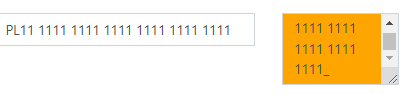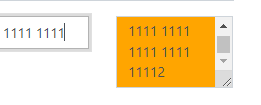# Immediate response to masked input

Hello together,

I have a function that writes the content of a masked input directly into an SP field. However, the copying only takes place when I click somewhere else, e.g. next to the field. Only then the function started. With all other Plumsail text fields, the function starts after each keyboard entry.
Is it possible to implement this for a masked input?
Thats my code:

fd.field('DUNS').\$on('change',function(value){
fd.field('DUNS_x002d_No_x0020_change').value = fd.field('DUNS').value;
})

I tried a different one but with the same result.

Best regards
Sternchen

Dear @Sternchen,
A bit more technical, but you can try this:

``````var elem = \$(fd.field('MaskedText1').\$el).find('input');
elem.bind("input", function(event){
console.log(event.currentTarget.value);
});
``````

Thank you.
I tried it like this and it seems like working.

var elem = \$(fd.field('DUNS').\$el).find('input');
elem.bind("input", function(event){
fd.field('DUNS_x002d_No_x0020_change').value = event.currentTarget.value;
});

1 Like

Hello @Nikita_Kurguzov
Seems like we are running into a problem with this solution.
I used the code and this happens:As you can see there is a '_' in the second sp field.
Or this.You shouldn´t been able to do this. How is it possibly to write something into the left field and not seeing it. But it gets copied into the right field. The left field only allows 24 characters so how can I ad a 25 character?
If I´m using blur instead of input it works fine. But the problem here is, that the function only starts when I click somewhere else.

Best regards
Sternchen

Dear @Sternchen,
Well, seems like the value is copied before the mask and conditions are applied. Does the second field have the same mask? If it doesn't help, perhaps, a similar check at the moment of copying might help - you can check length and not allowed symbols when copying the value, and only copy what's allowed.

The second field has no mask or anything. Only the first one has it.
I´ll try and check the length before copying it.
Do you have any idea how to check the length? The length of the masked input. Somehing like this ??

I tried it ike this:

var IBANMI = \$(fd.field('IBAN1').\$el).find('input');
IBANMI.bind("input", function(event){
if(fd.field('IBAN_x0020_change').length < fd.field('IBAN1').length){
fd.field('IBAN_x0020_change').value = event.currentTarget.value;
}
});

Dear @Sternchen.
Try it like this:

``````var elem = \$(fd.field('MaskedText1').\$el).find('input');
elem.bind('input', function(event){
if(event.currentTarget.value.length <= 24){
fd.field('IBAN_x0020_change').value = event.currentTarget.value;
}
});
``````